Olympiad Test: Odd One Out - 2

# Olympiad Test: Odd One Out - 2

Test Description

## 20 Questions MCQ Test Mathematics Olympiad Class 7 | Olympiad Test: Odd One Out - 2

Olympiad Test: Odd One Out - 2 for Class 7 2023 is part of Mathematics Olympiad Class 7 preparation. The Olympiad Test: Odd One Out - 2 questions and answers have been prepared according to the Class 7 exam syllabus.The Olympiad Test: Odd One Out - 2 MCQs are made for Class 7 2023 Exam. Find important definitions, questions, notes, meanings, examples, exercises, MCQs and online tests for Olympiad Test: Odd One Out - 2 below.
 1 Crore+ students have signed up on EduRev. Have you?
Olympiad Test: Odd One Out - 2 - Question 1

### Direction: In each problem, out of the five figures marked (1), (2), (3), (4) and (5), four are similar in a certain manner. However, one figure is not like the other four. Q. Choose the figure which is different from the rest.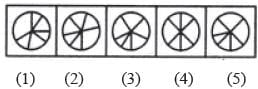Detailed Solution for Olympiad Test: Odd One Out - 2 - Question 1

Only figure (4) contains three diameters of the circle.

Olympiad Test: Odd One Out - 2 - Question 2

### Direction: In each problem, out of the five figures marked (1), (2), (3), (4) and (5), four are similar in a certain manner. However, one figure is not like the other four. Q. Choose the figure which is different from the rest.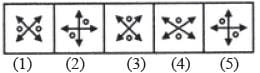Detailed Solution for Olympiad Test: Odd One Out - 2 - Question 2

In all other figures, the two double sided arrows intersect each other at right angles.

Olympiad Test: Odd One Out - 2 - Question 3

### Direction: In each problem, out of the five figures marked (1), (2), (3), (4) and (5), four are similar in a certain manner. However, one figure is not like the other four. Q. Choose the figure which is different from the rest.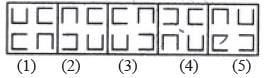Detailed Solution for Olympiad Test: Odd One Out - 2 - Question 3

Only in figure (1), two of the four elements are oriented in the same direction.

Olympiad Test: Odd One Out - 2 - Question 4

Direction: In each problem, out of the five figures marked (1), (2), (3), (4) and (5), four are similar in a certain manner. However, one figure is not like the other four.
Q. Choose the figure which is different from the rest.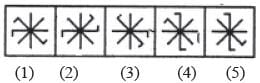Detailed Solution for Olympiad Test: Odd One Out - 2 - Question 4

All other figures can be rotated into each other.

Olympiad Test: Odd One Out - 2 - Question 5

Direction: In each problem, out of the five figures marked (1), (2), (3), (4) and (5), four are similar in a certain manner. However, one figure is not like the other four.
Q. Choose the figure which is different from the rest.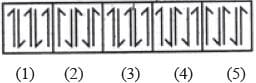Detailed Solution for Olympiad Test: Odd One Out - 2 - Question 5

In all other figures, each element is obtained by rotating the adjacent element through 180°.

Olympiad Test: Odd One Out - 2 - Question 6

Direction: In each problem, out of the five figures marked (1), (2), (3), (4) and (5), four are similar in a certain manner. However, one figure is not like the other four.
Q. Choose the figure which is different from the rest.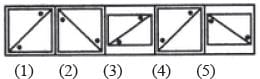Detailed Solution for Olympiad Test: Odd One Out - 2 - Question 6

The two dots lie on the same side of the diagonal only in figure (4).

Olympiad Test: Odd One Out - 2 - Question 7

Direction: In each problem, out of the five figures marked (1), (2), (3), (4) and (5), four are similar in a certain manner. However, one figure is not like the other four.
Q. Choose the figure which is different from the rest.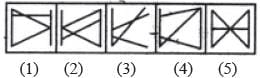Detailed Solution for Olympiad Test: Odd One Out - 2 - Question 7

All other figures contain five line segments while figure (3) contains four line segments.

Olympiad Test: Odd One Out - 2 - Question 8

Direction: In each problem, out of the five figures marked (1), (2), (3), (4) and (5), four are similar in a certain manner. However, one figure is not like the other four.
Q. Choose the figure which is different from the rest.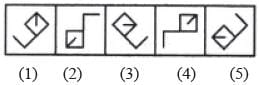Detailed Solution for Olympiad Test: Odd One Out - 2 - Question 8

The line segment inside the square is attached to the corner which lies opposite to the corner to which the outer L-shaped element is attached.

Olympiad Test: Odd One Out - 2 - Question 9

Direction: In each problem, out of the five figures marked (1), (2), (3), (4) and (5), four are similar in a certain manner. However, one figure is not like the other four.
Q. Choose the figure which is different from the rest.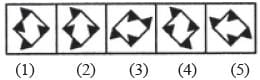Detailed Solution for Olympiad Test: Odd One Out - 2 - Question 9

Only in figure (4), each side of the square has one black triangle attached to it.

Olympiad Test: Odd One Out - 2 - Question 10

Direction: In each problem, out of the five figures marked (1), (2), (3), (4) and (5), four are similar in a certain manner. However, one figure is not like the other four.
Q. Choose the figure which is different from the rest.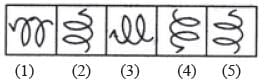Detailed Solution for Olympiad Test: Odd One Out - 2 - Question 10

All other figures can be rotated into each other.

Olympiad Test: Odd One Out - 2 - Question 11

Direction: In each problem, out of the five figures marked (1), (2), (3), (4) and (5), four are similar in a certain manner. However, one figure is not like the other four.
Q. Choose the figure which is different from the rest.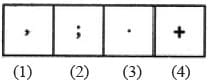Detailed Solution for Olympiad Test: Odd One Out - 2 - Question 11

All others are punctuation marks.

Olympiad Test: Odd One Out - 2 - Question 12

Direction: In each problem, out of the five figures marked (1), (2), (3), (4) and (5), four are similar in a certain manner. However, one figure is not like the other four.
Q. Choose the figure which is different from the rest.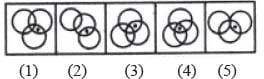Detailed Solution for Olympiad Test: Odd One Out - 2 - Question 12

Only in figure (4), the dot appears in the region common to all the three circles.

Olympiad Test: Odd One Out - 2 - Question 13

Direction: In each problem, out of the five figures marked (1), (2), (3), (4) and (5), four are similar in a certain manner. However, one figure is not like the other four.
Q. Choose the figure which is different from the rest.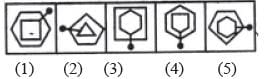Detailed Solution for Olympiad Test: Odd One Out - 2 - Question 13

Only in figure (4), the pin passes through a vertex of each one of the two elements.

Olympiad Test: Odd One Out - 2 - Question 14

Direction: In each problem, out of the five figures marked (1), (2), (3), (4) and (5), four are similar in a certain manner. However, one figure is not like the other four.
Q. Choose the figure which is different from the rest.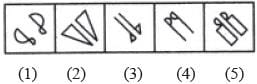Detailed Solution for Olympiad Test: Odd One Out - 2 - Question 14

All other figures contain a geometrical figure along with its mirror image.

Olympiad Test: Odd One Out - 2 - Question 15

Direction: In each problem, out of the five figures marked (1), (2), (3), (4) and (5), four are similar in a certain manner. However, one figure is not like the other four.
Q. Choose the figure which is different from the rest.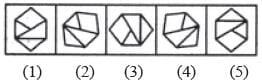Detailed Solution for Olympiad Test: Odd One Out - 2 - Question 15

All other figures can be rotated into each other.

Olympiad Test: Odd One Out - 2 - Question 16

Direction: In each problem, out of the five figures marked (1), (2), (3), (4) and (5), four are similar in a certain manner. However, one figure is not like the other four.
Q. Choose the figure which is different from the rest.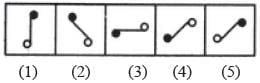Detailed Solution for Olympiad Test: Odd One Out - 2 - Question 16

Figure (c) is different from other figures.

Olympiad Test: Odd One Out - 2 - Question 17

Direction: In each problem, out of the five figures marked (1), (2), (3), (4) and (5), four are similar in a certain manner. However, one figure is not like the other four.
Q. Choose the figure which is different from the rest.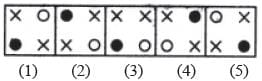Detailed Solution for Olympiad Test: Odd One Out - 2 - Question 17

In each one of the figures except figure (3), the two crosses (×) appear in the diagonally opposite corners.

Olympiad Test: Odd One Out - 2 - Question 18

Direction: In each problem, out of the five figures marked (1), (2), (3), (4) and (5), four are similar in a certain manner. However, one figure is not like the other four.
Q. Choose the figure which is different from the rest.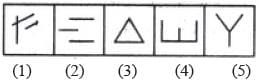Detailed Solution for Olympiad Test: Odd One Out - 2 - Question 18

Figure (4) is formed by four line segments while each one of the other figures is formed by three line segments.

Olympiad Test: Odd One Out - 2 - Question 19

Direction: In each problem, out of the five figures marked (1), (2), (3), (4) and (5), four are similar in a certain manner. However, one figure is not like the other four.
Q. Choose the figure which is different from the rest.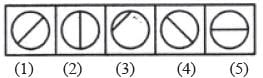Detailed Solution for Olympiad Test: Odd One Out - 2 - Question 19

Only in figure (3), the line segment is not a diameter of the circle.

Olympiad Test: Odd One Out - 2 - Question 20

Direction: In each problem, out of the five figures marked (1), (2), (3), (4) and (5), four are similar in a certain manner. However, one figure is not like the other four.
Q. Choose the figure which is different from the rest.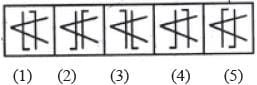Detailed Solution for Olympiad Test: Odd One Out - 2 - Question 20

Only in figure (4), both the parallel lines are bent in the same direction {i.e. towards the left).(Scan QR code)